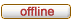# Horde3D

Next-Generation Graphics Engine
 It is currently 31.05.2020, 07:09

 All times are UTC + 1 hour

 Print view Previous topic | Next topic
Author Message
 Post subject: Vec3f operator== problemPosted: 23.05.2013, 08:06Joined: 26.08.2008, 18:48
Posts: 120
We found this bug when migrated our engine and tools to Visual Studio 2012.
Our geo files are 50%-200% larger after converting with the new ColladaConv.
The vertices are duplicated for each index. The problem is with Vec3f operator==

Code:
bool operator==( const Vec3f &v ) const
{
return (x > v.x - Math::Epsilon && x < v.x + Math::Epsilon &&
y > v.y - Math::Epsilon && y < v.y + Math::Epsilon &&
z > v.z - Math::Epsilon && z < v.z + Math::Epsilon);
}

For sufficiently large numbers "x - Math::Epsilon" or "x + Math::Epsilon" has exactly the same bit representation as "x" because the mantissa/significand is not large enough to represent the small change. In this case this function returns false even if this' value was exactly the same as v's value.

The difference between VS2008 and VS2012 is that the new version uses sse instructions while the old used standard floating point instructions.

I think the correct version should be:
Code:
bool operator==( const Vec3f &v ) const
{
return (x >= v.x - Math::Epsilon && x <= v.x + Math::Epsilon &&
y >= v.y - Math::Epsilon && y <= v.y + Math::Epsilon &&
z >= v.z - Math::Epsilon && z <= v.z + Math::Epsilon);
}

It also makes sense as the operator== and operator!= should satisfy that !(a==b) == (a!=b).

TopPost subject: Re: Vec3f operator== problemPosted: 24.05.2013, 12:59Joined: 21.08.2008, 11:44
Posts: 354
I think problem stems from SSE units being less accurate than FPU on some architectures. No idea about MSVC 2012, but on 64bit builds MSVC (2010) automatically enables SSE/SSE2 optimizations and uses scaler SSE instructions and XMM registers (Don't expect any noticeable speed up). Can you try this one too please? This generates smaller codeCode:
// Shouldn't Math::ZeroEpsilon instead being used here?

bool operator==( const Vec3f &v ) const
{
return (fabsf(x - v.x) <= Math::Epsilon &&
fabsf(y - v.y) <= Math::Epsilon &&
fabsf(z - v.z) <= Math::Epsilon);
}

EDIT: Changes are available at fastmath branch, commit 341318be15.

TopPost subject: Re: Vec3f operator== problemPosted: 24.05.2013, 18:19Tool Developer

Joined: 13.11.2007, 11:07
Posts: 1149
Location: Germany
Floating point comparing always sucks, as the preferable precision of comparison depends on the usage context most of the time.

There is also some code provided in the google test code provided here:
and some nice information here:
http://www.cygnus-software.com/papers/c ... floats.htm

If we found a solution that fits most of the test cases in a proper way (under VS2012 as well as under other compilers) I will commit that solution to the SVN.

TopPost subject: Re: Vec3f operator== problemPosted: 24.05.2013, 19:04Joined: 26.08.2008, 18:48
Posts: 120
Siavash wrote:
I think problem stems from SSE units being less accurate than FPU on some architectures. No idea about MSVC 2012, but on 64bit builds MSVC (2010) automatically enables SSE/SSE2 optimizations and uses scaler SSE instructions and XMM registers (Don't expect any noticeable speed up). Can you try this one too please? This generates smaller codeCode:
// Shouldn't Math::ZeroEpsilon instead being used here?

bool operator==( const Vec3f &v ) const
{
return (fabsf(x - v.x) <= Math::Epsilon &&
fabsf(y - v.y) <= Math::Epsilon &&
fabsf(z - v.z) <= Math::Epsilon);
}

It looks ok to me(same as "Comparing with epsilon – absolute error" in the doc attached by Volker), I will try on Monday at work. Also probably faster(if fabsf is implemented inline without a function call) as there are less branches.

Volker wrote:
Floating point comparing always sucks, as the preferable precision of comparison depends on the usage context most of the time.

There is also some code provided in the google test code provided here:
and some nice information here:
http://www.cygnus-software.com/papers/c ... floats.htm

If we found a solution that fits most of the test cases in a proper way (under VS2012 as well as under other compilers) I will commit that solution to the SVN.

Relative error comparisons seems complicated, I would choose Siavash's version.

TopPost subject: Re: Vec3f operator== problemPosted: 29.05.2013, 11:34Joined: 09.06.2012, 14:21
Posts: 12
IMO operator== should only check for exactly the same values. It is better to provide another function that uses epsilon comparison.

TopDisplay posts from previous: All posts1 day7 days2 weeks1 month3 months6 months1 year Sort by AuthorPost timeSubject AscendingDescending

 All times are UTC + 1 hour

#### Who is online

Users browsing this forum: No registered users and 1 guest

 You cannot post new topics in this forumYou cannot reply to topics in this forumYou cannot edit your posts in this forumYou cannot delete your posts in this forumYou cannot post attachments in this forum

Search for:
 Jump to:  Select a forum ------------------ Horde3D Usage    General Discussion    Support    Bug Reports    Asset Creation    Showcase    Advanced Rendering Techniques    Tools    Free Discussion Horde3D Development    Developer Discussion